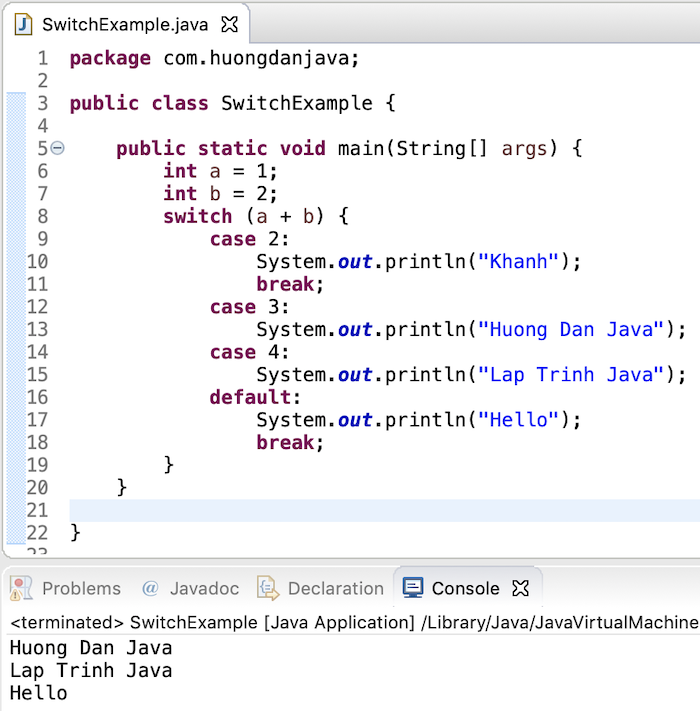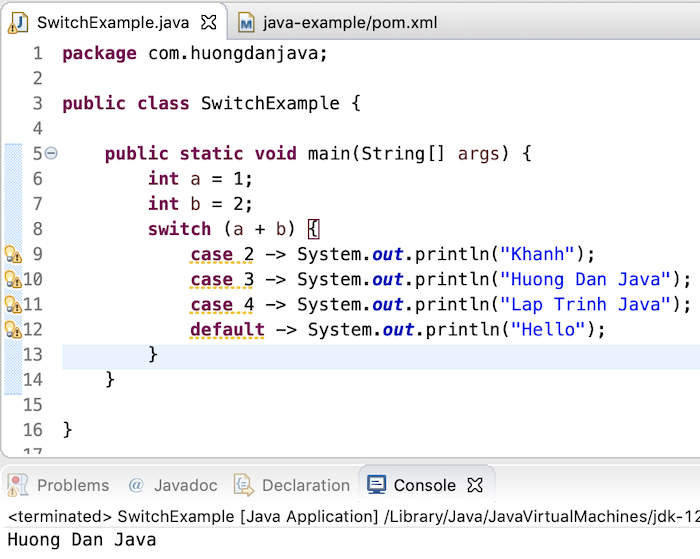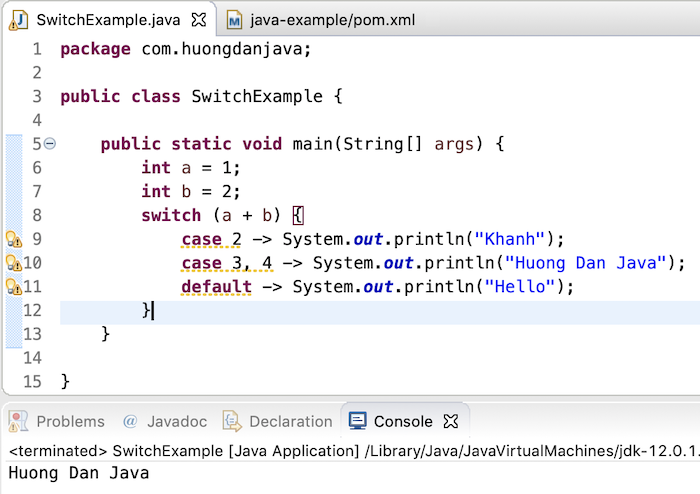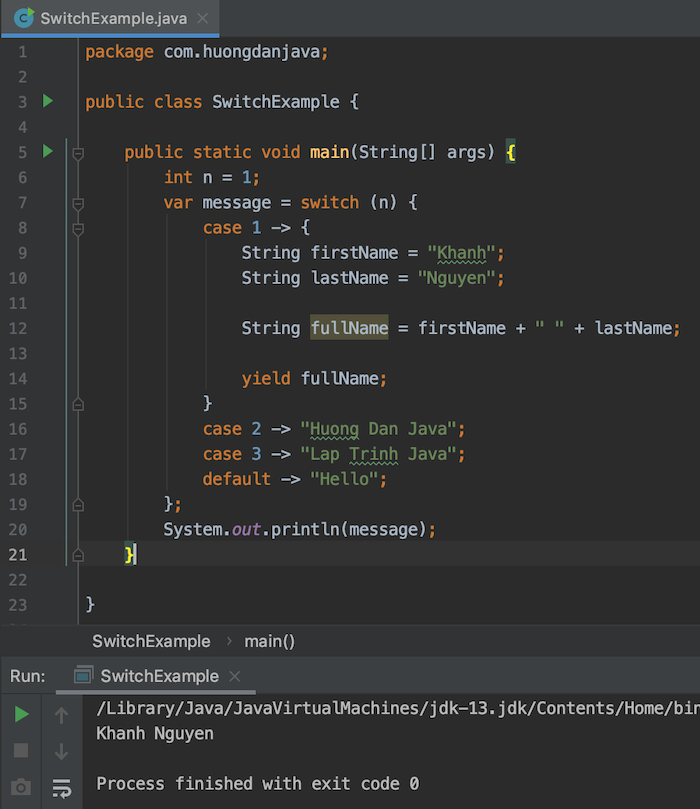Java enhancements for Switch statement since Java 12

When working with a switch statement, you can encounter some following problems:

• The first problem is that we forget to declare the break statement: this means that our code will not exit the switch statement after matching the first case but will continue the next case, for example:

Result:• The second problem is if we want in case the value of the variable with different values a and b, we will handle the same code. We will write the following code:

Or:

These ways of writing are inconvenient because duplicating the code or we sometimes can’t control it, where should we put a break statement?

• The third problem is that if you want to use a switch statement to determine the value of a variable, you must write the following:

To solve these problems, from Java 12, you can write a switch statement with the following improvements:

• For the first problem, Java 12 now supports us how to write a switch statement like lambda expression, for example. as:

Result:• For the second problem, you can rewrite the switch statement as follows:

Result:• As for the third problem, now Java 12 has supported us to return value with a switch statement as follows:

Result:If you want to add code to handle business logic in each case of the switch statement, then you need to upgrade to Java 13, then add the code and use the yield keyword to return the value you want. Example is as follows:

Result: